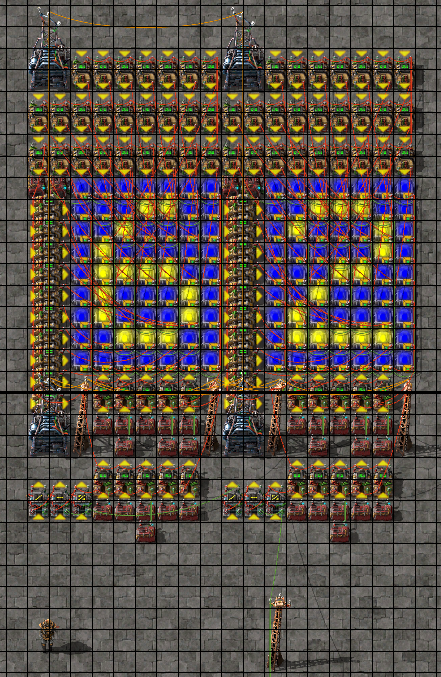# My 5x7 Dot Matrix Display for Factorio

2023-06-08 - A readable and tillable display I developed for my factories

## Introduction

A few months ago, I developed a 5x7 dot matrix display using combinators in Factorio. Most display examples you can find on the internet are hard to read 7 segments. I wanted to explore combinator circuits in factorio and decided to work out something more legible.

## The display

### How it works

There are a lot of combinators, but the whole behavior is not complex.

In the bottom left you have three arithmetic combinators:

• the rightmost one calculates the modulo of the input number and stores it in the N signal.
• the middle one subtracts N from the input number.
• the leftmost one divides the output of the second one by 10.

In the top left, surrounded by arithmetic combinators, there are two constant combinators which configure the colors of the display:

• The left one controls the foreground color.
• The right one controls the background color.

On the bottom, next to the three arithmetic combinators, you have a construction of 10 arithmetic combinators. Each is linked to one or two constant combinators. Depending on the value of the digit to display, which comes from the output of the modulo arithmetic combinator, one of these arithmetic combinators will relay the contents of its constant combinators to the display. These contents are a list of signals that will selectively light up the lamps composing the digit we need to display.

All the other arithmetic combinators at the top and on the left each control one of the lamps that form the matrix display. Each of these checks on a specific signal whether or not it should switch its lamp to the background color. The logic background/foreground is inverted because of the way lamps behave when they have two color inputs.

### Why it works

The display uses three important combinator features of factorio:

• The `Each` signal in the bottom left arithmetic combinators allows us to work with any input signal.
• The `Everything` signal in the bottom arithmetic combinators that evaluate digits allows us to forward a host of signals from the constant combinators.
• All the lamps get the foreground color signal, and the ones selected from the digit interpretation will also get the background color signal. There is an ordering to the color signals in factorio which gives priotity of one color over the over.

### How to wire it up

The input signal does not matter, but you need to have one and only one input signal and it needs to be a natural integer value. If you have multiple signals on your input wire, you need to setup an additional arithmetic combinator to filter a single signal to display.

Your input signal needs to be connected by a green wire to the input of the modulo combinator on the bottom left.

You can tile this design in order to display numbers with multiple digits, you just need to connect the output of the divider combinator of the lower order digit with the modulo combinator of the higher order digit with a green wire.## Conclusion

It is certainly possible to make a more compact build, but as long as it is tillable I do not really care. The way it currently works is simple to figure out and I will easily be able to patch in new characters if someday I want to display other things like letters of punctuation.

Here are some links for you: